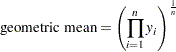### Arithmetic and Geometric Means

The arithmetic mean (more commonly called simply the mean) of the distribution of a random variable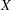is its expected value,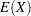. The arithmetic mean is the natural parameter of interest for a normal distribution because the distribution of the difference of normal random variables has a known normal distribution, and the arithmetic mean of a normal difference is equal to the difference of the individual arithmetic means. (No such convenient property holds for geometric means with normal data, with either differences or ratios.)

The usual estimate of an arithmetic mean is the sum of the values divided by the number of values: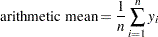The geometric mean of the distribution of a random variableis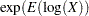, the exponentiation of the mean of the natural logarithm. The geometric mean is the natural parameter of interest for a lognormal distribution because the distribution of a ratio of lognormal random variables has a known lognormal distribution, and the geometric mean of a lognormal ratio is equal to the ratio of the individual geometric means. (No such convenient property holds for arithmetic means with lognormal data, with either differences or ratios.)

The usual estimate of a geometric mean is the product of the values raised to the power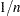, where n is the number of values: